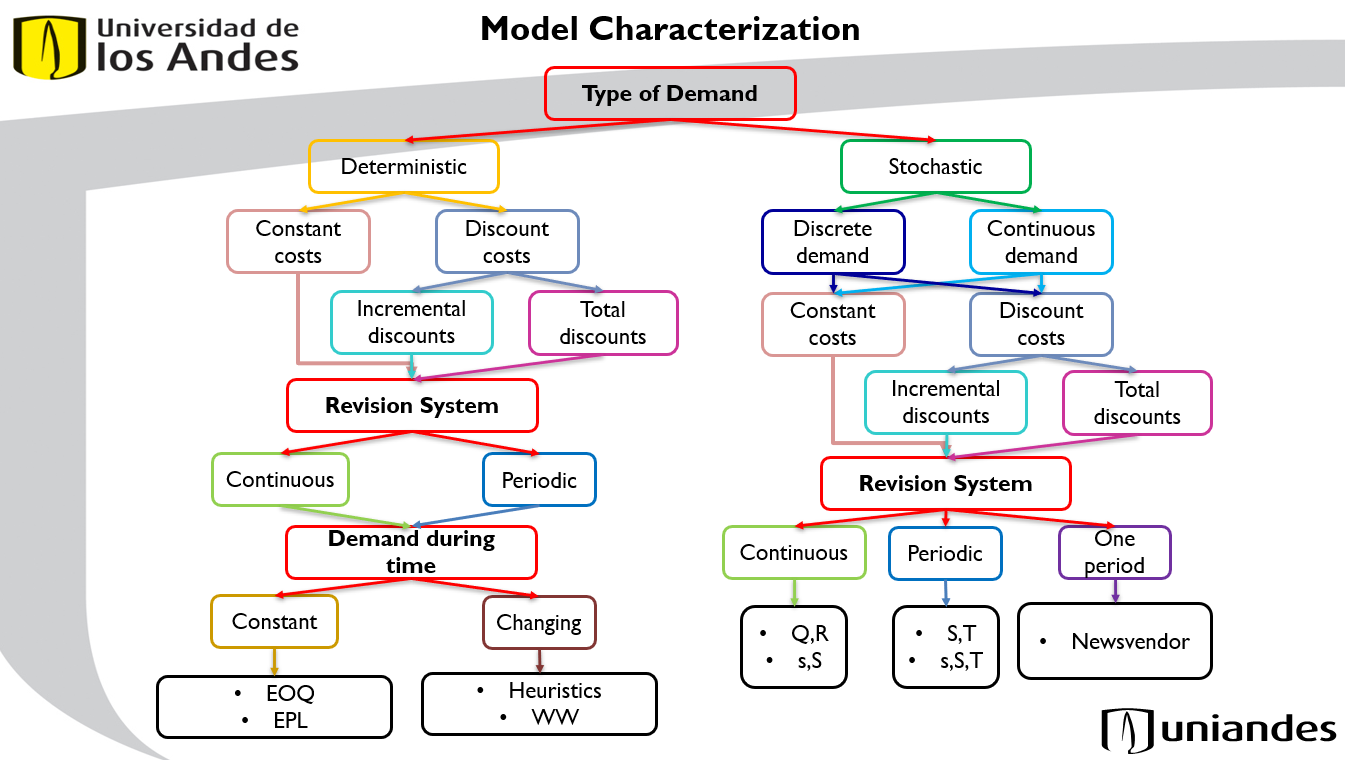# Chapter 1 Characterization

In order to develop models that can consider different situations, it is necessary to initially perform a characterization according to the main aspects of inventory control: Type of demand and Revision System. A characterization tree that considers these aspects is shown in Figure 1.1:Figure 1.1: Tree models characterization according to Type of demand and Revision System

For the construction of this tree, the categories described in the book “Production and Operation Analysis” (Nahmias and Olsen (2015)) were considered. In this book, the characteristics of an inventory system are mentioned: demand, lead time, revision time, excess demand and inventory changes. The thesis “Inventory Control and Demand Distribution Characterization” (Bai (2005)) similarly considers demand, lead time, excess inventory and shortage management (backorders/lost sales). In addition, he explains that the three key questions to be answered at the time of inventory control are:

1. How often should the status of the inventory level be reviewed?
2. When should the replenishment order be placed?
3. How many units should I order in each replenishment order?

The first question is related to the review system and timing, whether it is a continuous or periodic review system. If the review system is continuous, the review time is $$0$$, since the status of the inventory level is known at all times; if the review system is periodic, the review time is greater than $$0$$, and this determines how often the status of the inventory level should be reviewed. Depending on the review system, different inventory control models can be used. The second question is related to the cycle time between two orders placed. This will depend directly on the demand, since once an order is placed, it must be reordered after the inventory is depleted or a certain time has passed (which is also related to the demand). The third question refers to the number of units I should request from the supplier when placing an order. This depends on the demand and the cycle time. For the characterization of the tree, the characteristics of demand type and revision system are important. Therefore, in the characterization shown in Figure 1.1, the deterministic and stochastic demand types are considered. Then, it is divided into, in the case of stochastic demand, whether it is a discrete or continuous demand. After that, the type of costs are considered, between total or discounted, incremental or total costs. Following the costs, the Continuous and Periodic review systems are considered, and whether the demand is constant or variable (as would be the case of having different demands in the different periods to be analyzed) over time. Finally, the models that analyze each of the particular cases are shown. For example, for a deterministic demand, with constant costs, continuous review system and a constant demand over time, an EOQ model can be used for the case in which there is no production but finished product orders, or an EPL model for the case in which there is a production system.

### References

Bai, Liwei. 2005. “Inventory Control and Demand Distribution Characterization.” PhD thesis.
Nahmias, Steven, and Tava Lennon Olsen. 2015. Production an Operation Analysis. 7th ed. Long Grove, Il, United States: Waveland Press, Inc.